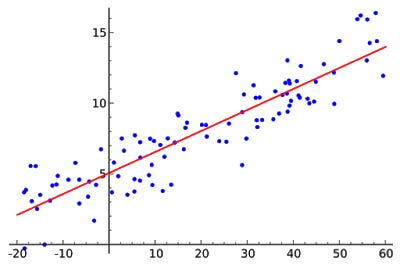# Linear Regression with Gradient Descent

### What is Regression?

According to Wikipedia,

In statistical modeling, regression analysis is a set of statistical processes for estimating the relationships among variables. It includes many techniques for modeling and analyzing several variables, when the focus is on the relationship between a dependent variable and one or more independent variables (or ‘predictors’). More specifically, regression analysis helps one understand how the typical value of the dependent variable (or ‘criterion variable’) changes when any one of the independent variables is varied, while the other independent variables are held fixed

### Linear Regression:

Y=mX+b

Linear regression is one of the basic way we can model relationships. Our model can be described as a line y=mx+b, m is the slope(to change the steepness and rotate about origin) of the line and b is the bias(y-intercept to move line up and down), x is the variable and y is the output at x. We can use linear regression to find linear relationship between data.

Linear Regression

A straight line approximates the relationship because we can’t use exponential or logarithmic function to model this kind of relationship. linear models are easy to understand and interpret. Although in real world most relationships aren’t linear. For that we have others models like Neural Networks which are universal function approximators. more on that later in future.

By changing m and b, we can find a line that fits best but how do we decide which is best? For that we calculate how much error our current m and b are adding up. Then we can change them in a way that gives us the best answer.

We have to find the best values for m and b such that we have minimum error(aka Cost). The error function here is MSE(Mean Squared Error). We initialize out m and b at random and then calculate error(cost). y_approx = m_current*x + b_current. We then add y_approx for every x taking their square. and we find mean by dividing by the number of points.

We can think m and b as knobs having so many combinations. Can’t we just brute force?? No, that would be very inefficient. We can do this by a method called Gradient Descent Optimization.

The Intuition behind Gradient Descent is that we move in a direction where the partial derivatives of m and b to Cost function are steepest.

We know that the derivatives gives us the rate of change, and zero derivative means we’re at either maxima or minima here we’ll move down here. To reach minima efficiently, we have to take optimal length of steps other wise we may reach at bottom very very late or we may not even reach there and over shoot. The length of steps is called Learning Rate.

It might happen that the cost function may not be non-convex. In this case we might end up on a local minima instead of a global minima. But in the case of linear regression, the cost function will always be a convex function.

Convex Vs. Non-convex

How we are updating our weights(m and b)? well using this equation

The partial derivatives of the gradients and they are used to update the values of m and b gamma is the learning rate which we need to specify. We then take steps and update our m and b accordingly until we reach a point where the cost function is minimum or until we want to stop.

Hope this was helpful!

Originally published at www.landofai.com.

Source: Deep Learning on Medium